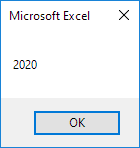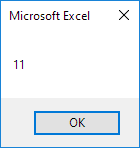# Date and Time

Learn how to work with dates and times in Excel VBA.

Place a command button on your worksheet and add the code lines below. To execute the code lines, click the command button on the sheet.

### Year, Month, Day of a Date

The following macro gets the year of a date. To declare a date, use the Dim statement. To initialize a date, use the DateValue function.

Code:

Dim exampleDate As Date

exampleDate = DateValue(“Jan 19, 2020”)

MsgBox Year(exampleDate)

Result:Note: Use Month and Day to get the month and day of a date.

To add a number of days to a date, use the DateAdd function. The DateAdd function has three arguments. Fill in “d” for the first argument to add days. Fill in 3 for the second argument to add 3 days. The third argument represents the date to which the number of days will be added.

Code:

Dim firstDate As Date, secondDate As Date

firstDate = DateValue(“Jan 19, 2020”)

secondDate = DateAdd(“d”, 3, firstDate)

MsgBox secondDate

Result:Note: Change “d” to “m” to add a number of months to a date. Place your cursor on DateAdd in the Visual Basic Editor and click F1 for help on the other interval specifiers. Dates are in US Format. Months first, Days second. This type of format depends on your windows regional settings.

### Current Date and Time

To get the current date and time, use the Now function.

Code:

MsgBox Now

Result:### Hour, Minute, Second

The get the hour of a time, use the Hour function.

Code:

MsgBox Hour(Now)

Result:Note: Use Minute and Second to get the minute and second of a time.

### TimeValue

The TimeValue function converts a string to a time serial number. The time’s serial number is a number between 0 and 1. For example, noon (halfway through the day) is represented as 0.5.

Code:

MsgBox TimeValue(“9:20:01 am”)

Result:Now, to clearly see that Excel handles times internally as numbers between 0 and 1, add the following code lines:

Dim y As Double

y = TimeValue(“09:20:01”)

MsgBox y

Result:Next Chapter: Events